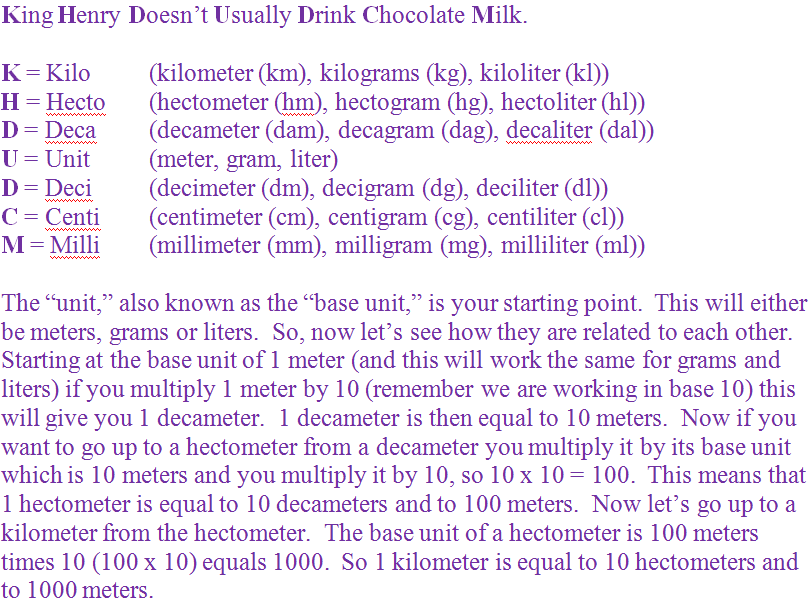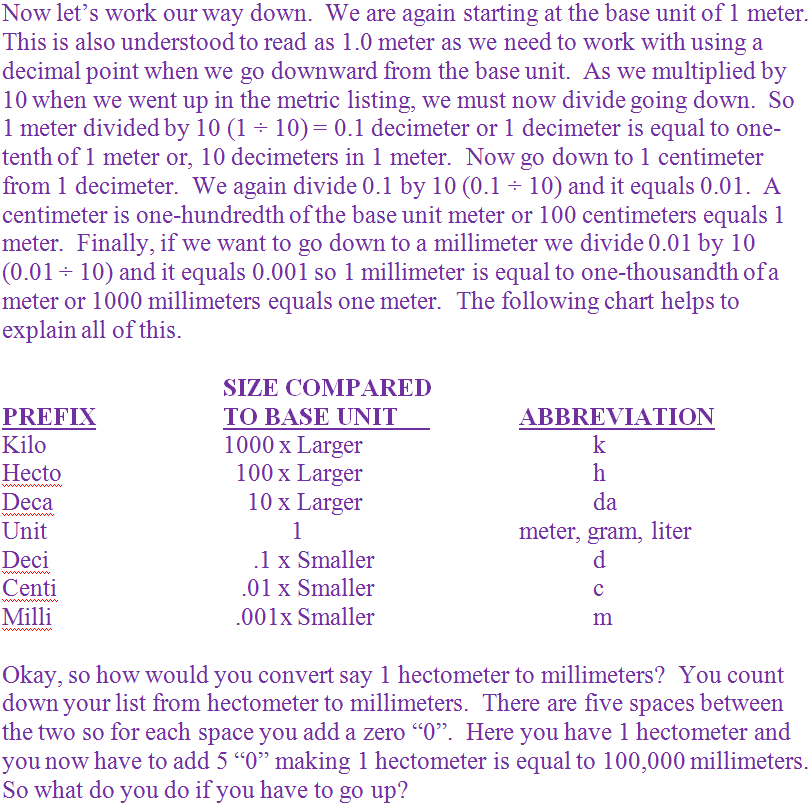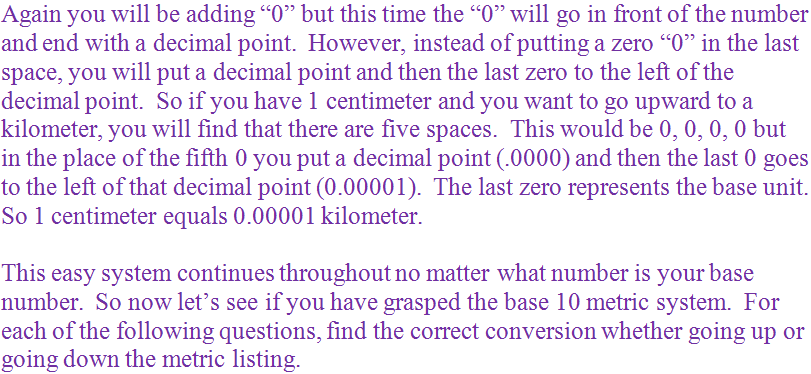# Math: Middle School: Grades 6, 7 and 8 Quiz - Measurement - The Metric System - Easy Conversions 1 (Questions)

This Math quiz is called 'Measurement - The Metric System - Easy Conversions 1' and it has been written by teachers to help you if you are studying the subject at middle school. Playing educational quizzes is a fabulous way to learn if you are in the 6th, 7th or 8th grade - aged 11 to 14.

It costs only \$12.50 per month to play this quiz and over 3,500 others that help you with your school work. You can subscribe on the page at Join Us

As you know, everything in life involves math in one form or another. Growing up in the United States, you have been taught the American or English system of math. However, most of the rest of the world does not use this system. Rather, they use the metric system.

You may not even realize it but you have already been exposed to the metric system. Pick up any can of vegetables or a bag of cookies and you will notice two different measurement amounts listed. You will see ounces or pounds and you will also see grams. When you buy soda, do you buy a pint of soda or a half gallon or soda or a gallon of soda? No, you buy soda in liters. Grams and liters are part of the metric system.

The metric system is really a very easy system to learn. It is easy because it works in base 10 conversions. This is true for each type of measurement such as for length or distance, known as meters, mass or weight, known as grams, and volume, known as liters. However, to be able to convert in the system, you do need to memorize the order of the system. To help you with that, memorize the following little phrase.

Have a look at the phrase and further explanation in purple below before tackling the questions.

Go straight to Quiz1. 1,000 g = ___ kg
 [ ] 1 [ ] 100 [ ] 1,000 [ ] 10,000
2. 1 cm = ___ mm
 [ ] 0.01 [ ] 0.1 [ ] 10 [ ] 100
3. 6 hl = ___ dl
 [ ] 600 [ ] 6,000 [ ] 6 [ ] .006
4. 12 m = ___ dam
 [ ] .12 [ ] 120 [ ] 1,200 [ ] 1.2
5. 8 l = ___ kl
 [ ] 0.08 [ ] 0.008 [ ] 800 [ ] 8,000
6. 120 m = ___ mm
 [ ] 120,000 [ ] 12,000 [ ] 1,200 [ ] 1.2
7. 25 g = ___ dg
 [ ] 25,000 [ ] 2,500 [ ] 250 [ ] 2.5
8. 7 kg = ___ g
 [ ] .007 [ ] 70 [ ] 700 [ ] 7,000
9. 94 cm = ___ dam
 [ ] 0.94 [ ] 0.094 [ ] 9.4 [ ] 940
10. 19 hg = ___ dg
 [ ] 190 [ ] 1,900 [ ] 19,000 [ ] 190,000Math: Middle School: Grades 6, 7 and 8 Quiz - Measurement - The Metric System - Easy Conversions 1 (Answers)
1. 1,000 g = ___ kg
 [x] 1 [ ] 100 [ ] 1,000 [ ] 10,000
As we are starting at the base unit of 1,000 grams, which is also understood to be 1,000.0, and we are moving up the metric list, we need to move the decimal point one space to the left for each space between the base unit and the kilogram. As there are three spaces the decimal point moves three spaces to the left turning 1,000.0 to 1.0. So, 1,000 grams (g) equals 1 kilogram (kg). Answer (a) is the correct answer
2. 1 cm = ___ mm
 [ ] 0.01 [ ] 0.1 [x] 10 [ ] 100
As we are moving down the metric list we will be adding zeroes “0”. There is one space between centimeters and millimeters so 1 centimeter (cm) equals 10 millimeters (mm). Answer (c) is the correct answer
3. 6 hl = ___ dl
 [ ] 600 [x] 6,000 [ ] 6 [ ] .006
As we are moving down the metric list we will be adding zeroes “0”. There are three spaces between hectoliters and deciliters so 6 hectoliters (hl) equals 6,000 deciliters (dl). Answer (b) is the correct answer
4. 12 m = ___ dam
 [ ] .12 [ ] 120 [ ] 1,200 [x] 1.2
As we are moving up from the base unit of 12 meters, it is understood that 12 meters is the same as 12.0. Moving up we must move the decimal point over one space to the left for each space we go up the metric list. The decameter is one space up from the meter so 12.0 becomes 1.2. 12 meters (m) equals 1.2 decameters (dam). Answer (d) is the correct answer
5. 8 l = ___ kl
 [ ] 0.08 [x] 0.008 [ ] 800 [ ] 8,000
As we are moving up from the base unit of 8 liters, it is understood that 8 liters is the same as 8.0. Moving up we must move the decimal point over one space to the left for each space we go up the metric list. The kiloliter is three spaces up from the liter so 8.0 becomes 0.008. 8 liters (l) equals 0.008 kiloliters (kl). (Remember the “0” before the decimal point represents the base unit.) Answer (b) is the correct answer
6. 120 m = ___ mm
 [x] 120,000 [ ] 12,000 [ ] 1,200 [ ] 1.2
As we are moving down the metric list we will be adding zeroes “0”. There are three spaces between meter and millimeters so 120 meters (m) equals 120,000 millimeters (mm). Answer (a) is the correct answer
7. 25 g = ___ dg
 [ ] 25,000 [ ] 2,500 [x] 250 [ ] 2.5
As we are moving down the metric list we will be adding zeroes “0”. There is one space between grams and decigrams so 25 grams (g) equals 250 decigrams (dg). Answer (c) is the correct answer
8. 7 kg = ___ g
 [ ] .007 [ ] 70 [ ] 700 [x] 7,000
As we are moving down the metric list we will be adding zeroes “0”. There are three spaces between kilograms and grams so 7 kilograms (kg) equals 7,000 grams (g). Answer (d) is the correct answer
9. 94 cm = ___ dam
 [ ] 0.94 [x] 0.094 [ ] 9.4 [ ] 940
As we are moving up the metric list we will be adding zeroes “0” to the left of the base number “94”. Remember, when counting the spaces up you place a decimal point in place of the last “0” and then place the last zero to the left of the decimal point to represent the base unit. So there are three spaces starting from 94.0 which then becomes .094 and then you add the final zero to the left of the decimal to get 0.094. So, 94 centimeters (cm) equals 0.094 decameters (dam). Answer (b) is the correct answer
10. 19 hg = ___ dg
 [ ] 190 [ ] 1,900 [x] 19,000 [ ] 190,000
As we are moving down the metric list we will be adding zeroes “0”. There are three space between hectograms and decigrams so 19 hectograms (hg) equals 19,000 decigrams (dg). Answer (c) is the correct answer